# Stability factor

Click here to go to our page on Normalized Determinate Function (NDF) (new for June 2017)

Here's a page on unstable amplifier examples.

Here's a cool approximation for GMAX that you need to know about

Here's a video on how car trailers can be made conditionally stable if you put too much weight behind the axle.  Stability is relevant in many fields, and should be a consideration in all design activity. You should never tailgate a car or truck on the highway, and especially never tailgate a car or truck that is pulling a trailer.   The video perfectly illustrates the idea of conditional stability. Treat the system delicately and it behaves (like when you RF-probe an unstable device ant it sees a broadband match).  If the system sees an unusual but perfectly normal event, you could be in a world of hurt.

Back in 2006, we contacted  Microwave Hall-of-Famer John Rollett regarding his contribution to the art of microwaves (the stability factor, "K"). Please note that Mr. Rollett's last name rhymes with wallet; some engineers mistakenly try to give it French-sounding pronunciation. Yet another fact that you won't learn anywhere else but Microwaves101!

Below is John's perspective on the stability factor that bears his name:

"In 1955 I joined a team of scientists set up in London to make germanium transistors. As the junior member, I was given the job (which no one else wanted) of devising methods of assessing their capabilities. It emerged that "f/T" was the preferred indicator of useful bandwidth, and I designed more than one method of measuring this, and also became interested in other transistor parameters, resulting in the stability factor. In 1963, I joined what is now British Telecomms, where a team was developing and manufacturing highly reliable silicon transistors for the first transistorised transatlantic cable amplifiers. All these transistors were measured in my high-frequency gain (f/T) apparatus. At about the same time the stability factor began to find application in microwave circuits, something I had not envisaged! My interests then turned to active filters, and eventually to speech processing (synthesis, recognition, compression - GSM), far removed from microwaves. I retired in 1990.

It is interesting that a concept which was developed largely in the abstract, and with no specific applications in mind, should turn out to be useful (as indeed I hope it is).

My opinion about the application of the stability factor is not worth much, as it was developed from theoretical principles, and I never used it on a real amplifier, nor did I know of anyone who had, other than reading the literature.

I really have nothing useful to say about the stability factor, as for me it was mainly an exciting theoretical development, inspired by Sam Mason (who started off a paper in the IEEE "In a hundred years the earth will be covered with paper to a depth of eighty feet, and we shall all be as bald as billiard balls." Great man!). It was a pleasant surprise to see that it had useful applications. It also provided a main topic of my PhD thesis, where I also demonstrated that it could be measured directly, without being calculated from two-port parameters."

Along with this fine page on stability factor, we now have a download spreadsheet for calculating stability factor and available gain (and many other parameters) from vector S-parameters that you can get from manufacturers' data sheets! It's called S-Parameter Utilities 101, here's a page that explains how to use it.

Stability - what is it?

Passive versus active devices

Conditional stability versus unconditional stability

Rollett's stability factor (K-factor)

Maximum gain

Available gain

Stable gain

Other measures of stability

Mu1 and Mu2

B1

Examples of an unstable amplifiers you could buy (separate page)

### Stability - what is it?

Before we get into this subject, there are upwards of 1000 microwave eggheads out there who will tell you "looking at K-factor isn't enough to be 100% certain that your amplifier won't oscillate, you need to examine every possible loop for origin encirclements of right hand poles yadadadada..." to which we say, "shut up". When you are picking out an amplifier, always look at its K-factor, ideally from zero Hertz (DC) to the maximum frequency of oscillation Fmax of the device that it is based on, at steps no larger than 1 GHz. If you are a power amp designer, there is a lot more you should be looking at, but we won't cover all of that here.

Stability, in referring to amplifiers, refers to an amplifier's immunity to causing spurious oscillations. The oscillations can be full power, large-signal problems, or more subtle spectral problems that you might not notice unless you carefully examine the output with a spectrum analyzer, one Hertz at a time! Unwanted signals may be nowhere near your intended frequency but will wreak system havoc all the same. In another extreme, instability outside your band may drop the gain of your amplifier by 20 dB inside the band, which should get your attention. These types of problems are ones that you'd rather avoid.

A passive device contains nothing that could add energy to your signal. The first law thermodynamics, conservation of energy, implies that a passive device can't oscillate. An active device is one in which an external energy source is somehow contributing to the magnitude of one or more responses. Learn more about basic network theory here.

Conditional stability refers to a network that is stable when its input and output "see" the intended characteristic impedance Z0 (usually 50 ohms, sometimes 75 ohms), but if your application presents a mismatch, there is a region of either source or load impedances that will definitely cause it to oscillate (see our page on the Smith chart for more discussion of impedance). The phrase "potentially unstable" refers to the same condition, the difference is the same as the question of whether a glass is half-full or half empty.

Unconditional stability refers to a network that can "see" any possible impedance on the Smith chart from the center to the perimeter (up to gamma=1.0) at any phase angle. Gamma < 1 means that the real part of the impedance is positive. Note that any network can oscillate if it sees a real impedance that is negative, so if your system goes outside the normal Smith chart all bets on stability are off.

Stability has to be separately evaluated at all frequencies where the amplifier could potentially oscillate. This is generally up to the Fmax of the technology. For power PHEMT parts, stability is not a problem past 50 GHz or so.

### Rollet's stability factor (K-factor)

John Rollett's 1962 IRE paper titled Stability and Power-Gain Invariants of Linear Twoports forever links him to the stability factor, K, of an amplifier. For this reason we've put him in our Microwave Hall of Fame! From this one scalar dimensionless quantity you get the most valuable measure of stability for a given frequency.

Although a lower case is sometimes used, we'll follow Gonzalez and use upper case K whenever we refer to stability factor on this site. Thanks, Andy!

Whether you are designing an amplifier, or merely picking out an amplifier from vendor data sheets to use in a subsystem, you should always consider stability across frequency. Rollett's stability factor is a one way to get an indication of whether you'll have a problem or not.

K-factor that is greater than one tells you that your amplifier is unconditionally stable. If K is less than 1, you may have a problem. Below is the equation for K-factor (in two parts):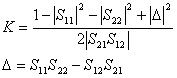Thanks to Ed we corrected the top equation on April 17, 2007! The plus sign was previously shown incorrectly as a minus sign.

Note that K-factor is only defined for two-port networks. That's why you don't see "S31", "S33", etc. in the equation.

A good amplifier designer must pay attention to available gain (GMAX, see below) and K-factor, being cognizant of the fact that high stability comes at the price of low available gain. The best amplifiers usually have K-factor as low as 1.5 in the band.

Here's a page on unstable amplifier examples which you should read before you ever buy an RF amplifier!

### Maximum available gain versus maximum stable gain

The maximum available gain (sometimes called MAG, sometimes called GMAX) of a device is only defined where K is greater than one. Algebraically, this is because the term under the square-root becomes negative for values of K less than 1. Another way to look at it is that maximum available gain is infinite. Infinite gain means oscillator.

The maximum stable gain (MSG) of a device is defined when maximum available gain is undefined (K<1). It is merely the ratio of mag(S21)/mag(S12). Under no circumstances should you try to tease more than this amount of gain from a conditionally stable device. Better yet, you should try to stabilize the device using resistive components, until K is greater than 1, then you can optimize the matching networks for all the gain you want.

### Calculating GMAX

Looking at available gain (GMAX) is also helpful when you are looking for potential instabilities. The equation below shows how GMAX is calculated from stability factor K and the forward and reverse transmission coefficients: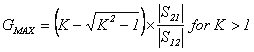Note that GMAX is only defined for two-port networks.

Available gain is undefined when K is less than one. That's when the square root of (K^2-1) becomes imaginary.

We found out the hard way that the GMAX equation can give you a big problem when K gets really big, which it often does. Our S-parameter Utilities spreadsheet (available in the download area) calculates GMAX, and whenever K became greater than about 20,000,000, GMAX would become zero in the spreadsheet, which makes no physical sense. It's because within the expression we are subtracting two very large numbers that only differ by a small amount, and Excel only maintains 15 digits of accuracy. So how does Agilent ADS deal with this problem, short of doubling the precision of the number?

We found empirically that the equation below gives the proper result with no worries about K being large (but is inaccurate when K is not large, so watch out!). In our free download S-parameter Utilities spreadsheet we substitute this whenever K is greater than 1,000,000: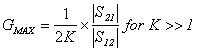If you are interested in proving why this approximation is valid, we believe you'll need to perform a Taylor series expansion of the original equation and then allow K to go to infinity. We'll let some microwave professor assign that math problem for extra credit, we just know that the equation works correctly when K is greater than 1,000,000, having checked it against some very expensive EDA software.

Update August 15 2008: here's the math behind the approximation, thanks to Raj! There's a mathematical approximation that comes into play, you can read about it on Wikipedia but here is the important part: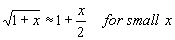Applying this approximation to the formula for GMAX when 1/K^2 is very small (K is very large), we see that: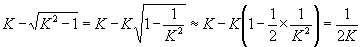and hence:Thanks, Raj!

Update, July 2016: you don't need to approximate the GMAX calculation, you just need to re-arrange it.  Thanks to Hadrien for pointing this out.  In the original equation,Replace "K-sqrt(K^2-1)" with "1/(K+sqrt(K^2-1))"

Here is the derivation:

K-sqrt(K^2-1) = (K-sqrt(K^2-1))*(K+sqrt(K^2-1))/(K+sqrt(K^2-1))

(K-sqrt(K^2-1))*(K+sqrt(K^2-1)) = K^2-(K^2-1) = 1

(K-sqrt(K^2-1))*(K+sqrt(K^2-1))/(K+sqrt(K^2-1)) = 1 / (K+sqrt(K^2-1))

We tried the calculation, it works great and doesn't not blow up for large K.  Maybe a lot of textbooks should be re-written to adopt the new expression.  As Hadrien points out, for numerical solutions of fixed precision, adding large number is good, subtracting them is bad.

### Other measures of Stability

There are many other measures of stability, but K-factor should remain the go-to measure for designers.

#### Mu1 and Mu2

Mu1 measures the radius from the center of the Smith Chart (Z0) to the nearest unstable point in the output plane. Mu2 does the same thing for the input plane. Obviously you want both of them to be greater than one to move the unstable point off of the Smith Chart. The larger Mu1 and Mu2 are, the more margin your design has to variations in processing. Mu1 and Mu2 are only defined for two-port networks (just like Gmax and K-factor).

The Mu factors tell you something that K-factor does not. They tell you which side of your circuit is the likely culprit when it goes unstable. However, if K is much greater than one, the Mu factors will also be much greater than one.

#### B1

B1 is another stability factor similar to K. Be honest, does anyone ever use this?

B1 = 1+|S11|2-|S22|2-|Δ|2

where Δ is defined in the K-factor calculation.

B1 should be greater than 0. This measurement like all of the others on this page) is only defined for two-port networks.

### Examples of unstable amplifiers you could buy

Moved to a new page.

Author : Unknown Editor